© 2021 Jasper van Baten, AmsterCHEM

## Examples:

### The basics

Let's start with the basics. Each Unit Operation requires a `Configure` function and a `Calculate` function. Each of these functions takes a `unit` argument. During `Configure`, one can add parameters, ports and reports, using `add_parameter`, `add_port` and `add_report`.

During `Calculate`, the ports can be obtained from `unit.ports[`<name>`]`, that is, the objects connected to the ports are obtained like that. If a ports is not connected, it will not appear in the `unit.ports` dictionary. Ports can only be left unconnected, if `optional=True` was specified as argument to `add_port`. Values can be obtained from `FEED` ports, while `PRODUCT` ports must be fully specified.

Similarly, parameters are available during `Calculate` from `unit.parameters[`<name>`]`. The `value` of an `INPUT` parameter is known at the start of `Calculate`, whereas the value of an `OUTPUT` parameter must be set during `Calculate`.

Reports are available from `unit.reports[`<name>`]`; reports can subsequently be written using e.g. `print` with the `file` argument set to the report.

def Configure(unit): #add a feed and product unit.add_port("Feed",unit.PortType.MATERIAL,unit.PortDirection.FEED) unit.add_port("Product",unit.PortType.MATERIAL,unit.PortDirection.PRODUCT) #add a heat duty parameter WATT=unit.Dimension.KILOGRAM*unit.Dimension.METER**2/unit.Dimension.SECOND**3 unit.add_parameter("Heat Duty",unit.ParameterType.REAL,unit.ParameterMode.INPUT,default=0,dimension=WATT) def Calculate(unit): #copy Feed to Product, apply heat duty fd=unit.ports["Feed"] duty=unit.parameters["Heat Duty"].value if fd.flow_rate==0 and duty!=0: raise RuntimeError('Zero feed flow rate; cannot apply duty') h=fd.get_property('enthalpy') #molar enthalpy if (duty!=0): h+=duty/fd.flow_rate unit.ports["Product"].set(x=fd.x,p=fd.p,flow_rate=fd.flow_rate,enthalpy=h)

During configure, two `MATERIAL` ports are added; a `FEED` called "Feed", and a `PRODUCT` called "Product".

### Calculating thermo-physical properties

The example uses `fd.flow_rate`, `fd.x` and `fd.t` to access total flow rate, mol/s, overall composition, mol/mol, and temperature, K. Material streams, or rather phase equilibria in general, are defined by composition, temperature, pressure, phase presence, and for each phase, composition, temperature, pressure and phase fraction. These properties can be used directly.

Other properties can be calculated using `get_property`; for a `FEED` `MATERIAL` and `PhaseEquilibrium`, get_property delivers equilibrium properties; for a `Phase`, `get_property` delivers single phase properties, for a `PhasePair`, `get_property` delivers two-phase properties. The lists of equilibrium properties, single phase properties and two phase properties can be obtained from a `MATERIAL` stream using `phase_equilibrium_properties`, `phase_properties` and `phase_pair_properties`. Alternatively, a `Phase` returns single the list of available single phase properties as `properties`, and a phase pair returns the list of two phase properties as `properties`.

Pure compound constant properties can be obtained from a `Compound`; the list of compounds is exposed by a `MATERIAL` stream's `compounds` member. Constant properties can be obtained from a `Compound` using `get_constant`, and temperature dependent properties can be obtained using `get_temperature_dependent_property`; the lists of these properties are available from `constant_properties` and `temperature_dependent_properties` for a `Compound`, or, equivalently, `constant_compound_properties` and `temperature_dependent_compound_properties` from a `MATERIAL` stream. One can directly obtain `name`, `molecular_weight`, `formula` and `cas_registry_number` from a compound.

#Python CAPE-OPEN Unit Operation script def Configure(unit): #example: add a feed unit.add_port("Feed",unit.PortType.MATERIAL,unit.PortDirection.FEED) def Calculate(unit): #example: calculate physical properties fd=unit.ports["Feed"] #some compound properties compound=fd.compounds; #first compound print("For compound",compound.name) print("Molecular weight",compound.molecular_weight,"gr/mol") print("Chemical formula",compound.formula) print("Critical temperature",compound.get_constant('criticalTemperature')) for t in [300,350,400]: print("Vapor pressure at",t,"K = ",compound.get_temperature_dependent_property('vaporPressure',t),"Pa") #one can get multiple properties as a tuple by specifying a list of property names [Psat,dPsatdT]=compound.get_temperature_dependent_property(['vaporPressure','vaporPressure.Dtemperature'],300) #equilibrium properties for the feed stream print("Feed temperature",fd.t,"K") print("Feed pressure",fd.p,"Pa") print("Feed composition",fd.x,"mol/mol") print("Feed vapor fraction",fd.vapor_fraction,"mol/mol") #and rate print("Feed rate",fd.flow_rate,"mol/s") #other properties require calculation print("Feed enthalpy",fd.get_property('enthalpy'),"J/mol") #multiple properties can be calculated in one call, returned as tuple [h,s,v]=fd.get_property(['enthalpy','entropy','volume']) #the same calculations can be performed on any phase equilibrium eq=fd.create_phase_equilibrium(fd.x,'pressure',2e5,'enthalpy',h) #adiabatic re-flash of feed at 2 bar eq=fd.create_phase_equilibrium(x=fd.x,p=2e5,enthalpy=h) #same thing, alternative syntax print("Equilibrium temperature",eq.t,"K") print("Equilibrium pressure",eq.p,"Pa") print("Equilibrium composition",eq.x,"mol/mol") print("Equilibrium vapor fraction",eq.vapor_fraction,"mol/mol") #other properties require calculation print("Equilibrium entropy",eq.get_property('entropy'),"J/mol/K") #multiple properties can be calculated in one call, returned as tuple [h,s,v]=eq.get_property(['enthalpy','entropy','volume']) #one can iterate over phases of feed or calculated equilibrium and # obtain single phase properties: for p in fd.phases: print(p.name,'temperature',p.t,'K') print(p.name,'pressure',p.p,'Pa') print(p.name,'composition',p.x,'mol/mol') print(p.name,'phase fraction',p.phase_fraction,'mol/mol') #other single phase properties must be calculated print(p.name,'enthalpy',p.get_property('enthalpy'),'J/mol') #again mutiple properties can be calculated in one go [visc,density]=p.get_property(['viscosity','density']) #for two phase properties, two phases can be combined in a # PhasePair object eq=fd.create_phase_equilibrium(x=fd.x,p=1e5,vaporFraction=0.5) #flash results vapor and liquid vapor_liquid=fd.create_phase_pair(eq.phases,eq.phases) print('surface tension',vapor_liquid.get_property('surfaceTension'),'N/m') #non-equilibrium phases can be created from a PhaseType or directly vapor1=fd.phase_types.create_phase(t=300,p=2e5,x=fd.x) vapor2=fd.create_vapor_phase(t=300,p=2e5,x=fd.x) print('vapor 1 density',vapor1.get_property('density'),'mol/m3') print('vapor 2 density',vapor2.get_property('density'),'mol/m3')

### Units of measure

In example 1, an `INPUT`, `REAL` parameter "Heat duty" is added that has the dimension WATT.

WATT is constructed from the base dimensions as kg*m2/s3. All real numbers in CAPE-OPEN are exchanged in pure SI units. For real parameters and information ports you get to decide on the dimensionality of the number; for properties on `MATERIAL` ports, the dimension is predefined. In many cases CAPE-OPEN allows a choice between specific and molar units; the Python Unit Operation always selects molar units. So flow rates are in mol/s, compositions are in mol/mol, density is in mol/m3, enthalpy in J/mol, etc.

For temperature differences and pressure differences, conversions from guage pressure and Celcius or Fahrenheit should not take into account the offset. For such quantities, pass `difference=True` in addition to the `dimension` argument.

Example 3 shows some ways to setup units of measure. A `Calculate` method is not provided in example 3.

def Configure(unit): #some unit of measure examples JOULE=unit.Dimension.KILOGRAM*unit.Dimension.METER**2/unit.Dimension.SECOND**2 WATT=JOULE/unit.Dimension.SECOND HERTZ=1/unit.Dimension.SECOND NEWTON=unit.Dimension.KILOGRAM*unit.Dimension.METER/unit.Dimension.SECOND**2 PASCAL=NEWTON/unit.Dimension.METER**2 #a heat duty parameter unit.add_parameter("Heat Duty",unit.ParameterType.REAL,unit.ParameterMode.INPUT,default=0,dimension=WATT) #a pressure drop parameter unit.add_parameter("Pressure drop",unit.ParameterType.REAL,unit.ParameterMode.INPUT,default=0,dimension=PASCAL,difference=True) #dimensions are also passed to information streams unit.add_port("Frequency",unit.PortType.INFORMATION,unit.PortDirection.PRODUCT,dimension=HERTZ)

### Validation

CAPE-OPEN unit operations are validated prior to calculation, and the PME is required to validate a Unit Operation after any change in configuration (not including changes to values on feed or product streams, provided the configuration of the streams does not change).

Unit operations do not need to be validated in case Calculate is called multiple times and the configuration is not changing, for example if the Unit Operation is in a recycle or during a parametric study.

Python Unit Operations may optionally implement a Validate function. A Validate function can raise an exception in case the configuration of the Unit Operation is not valid, or in case the streams connected to the Unit Operation have a configuration that is not suitable for the Unit Operation. In addition, the Unit Operation can store data during validation, that pertains to the configuration of the streams. After all, the PME must revalidate the Unit Operation in case this configuration changes. Such data typically includes information on phase or compound mapping.

During `Validate`, data can be stored in the `validation_data` dictionary, and then the data will be available during `Calculate`. The Unit Operation must not store any reference to external objects, such as streams, compounds, etc, directly or indirectly. Such objects cannot be referenced between calculations or validations. If the Unit Operation stores external references, behaviour is unpredictable.

The following example shows a water separator. This unit copies the feed stream to the product stream after taking out any water. The water is placed on a separate product stream called "Water". The example features a Validate function that locates water, first by CAS registry number, then by chemical formula, and if all fails, by name. Exception handling is used in case compounds are present that have no CAS registry number or chemical formula. If water is not found, validation fails. Otherwise the index of water in the compound slate is stored in `validation_data` and used during `Calculate`. For vector math, `numpy` is used. Note that no special care is taken in this example for zero flow rate of the Product stream, which will lead to a division by zero.

import numpy as np #Example water separator def Configure(unit): unit.add_port("Feed",unit.PortType.MATERIAL,unit.PortDirection.FEED) unit.add_port("Product",unit.PortType.MATERIAL,unit.PortDirection.PRODUCT) unit.add_port("Water",unit.PortType.MATERIAL,unit.PortDirection.PRODUCT) def Validate(unit): water_index=-1 compounds=unit.ports["Feed"].compounds #try by cas number for i in range(0,len(compounds)): try: #in case of compound without CAS number if compounds[i].cas_registry_number=='7732-18-5': water_index=i break except: pass #try by chemical formula if water_index==-1: for i in range(0,len(compounds)): try: #in case of compound without formula if compounds[i].formula=='H2O': water_index=i break except: pass #try by name if water_index==-1: for i in range(0,len(compounds)): if compounds[i].name.lower()=='water': water_index=i break if water_index==-1: raise RuntimeError('water not found') #store water index for Calculate unit.validation_data['water_index']=water_index def Calculate(unit): fd=unit.ports["Feed"] #compound flow rates comp_rates=np.array(fd.x)*fd.flow_rate #take water out water_index=unit.validation_data['water_index'] water_rate=comp_rates[water_index] comp_rates[water_index]=0 #set product, at feed t and p product_rate=comp_rates.sum() unit.ports["Product"].set(flow_rate=product_rate,x=comp_rates/product_rate,p=fd.p,t=fd.t) #set water stream at feed t and p x_water=np.zeros(len(comp_rates)) x_water[water_index]=1 unit.ports["Water"].set(flow_rate=water_rate,x=x_water,p=fd.p,t=fd.t)

Your custom implementation of `Validate` is called after all built-in validation has already passed. Built in validation checks that all ports that are not marked as `optional=True` are connected, and that all input parameters not marked with `optional=True` are specified, and that the values are valid (e.g. not violating imposed minimum and maximum values). Then it will check that the compounds found on each of the connected material streams are consistent. For some PMEs this is always the case. Other PMEs allow you to define streams with different thermodynamic subsystems, and therefore different compounds, and will allow you to connect such streams to a unit operation. By default, the Python Unit Operation will not validate in such a case.

Sometimes it can be useful to allow for this. Consider a heat exchanger with a hot and a cold stream, or more generally, a stream 1 and stream 2. There is no particular reason that stream 1 and stream 2 should have the same compound slates. One side of your heat exchanger may be a process stream, while the other side may be steam for example. Consider the following configuration:

Now, connecting Feed 1 consistently with Product 1, and Feed 2 consistently with Product 2, but Feed 1 and Feed 2 inconsistently, will fail to validate with the following message:

`material objects connected to ports 'Feed 2' and 'Feed 1' do not have same compounds`

This behaviour can be changed by setting `unit.allow_inconsistent_compounds=True` in `Configure`. At this point you should take care of validation of consistent compound slates where required yourself.

#Heat Exchanger, Counter-current, Max heat exchange def Configure(unit): #material ports unit.add_port("Feed 1",unit.PortType.MATERIAL,unit.PortDirection.FEED) unit.add_port("Product 1",unit.PortType.MATERIAL,unit.PortDirection.PRODUCT) unit.add_port("Feed 2",unit.PortType.MATERIAL,unit.PortDirection.FEED) unit.add_port("Product 2",unit.PortType.MATERIAL,unit.PortDirection.PRODUCT) #allow inconsistent compound slates unit.allow_inconsistent_compounds=True def Validate(unit): compoundSlate1=[comp.name for comp in unit.ports["Feed 1"].compounds] compoundSlate2=[comp.name for comp in unit.ports["Product 1"].compounds] if compoundSlate1!=compoundSlate2: raise RuntimeError('Feed 1 and Product 1 do not have the same compounds') compoundSlate1=[comp.name for comp in unit.ports["Feed 2"].compounds] compoundSlate2=[comp.name for comp in unit.ports["Product 2"].compounds] if compoundSlate1!=compoundSlate2: raise RuntimeError('Feed 2 and Product 2 do not have the same compounds') def Calculate(unit): fd1=unit.ports["Feed 1"] fd2=unit.ports["Feed 2"] pd1=unit.ports["Product 1"] pd2=unit.ports["Product 2"] if fd1.flow_rate==0: #zero flow case, no heat transfer possible; bring stream 1 to feed 2 temperature pd1.set(x=fd1.x,p=fd1.p,flow_rate=fd1.flow_rate,t=fd2.t) # do a PH flash in case stream 2 is a pure compound pd2.set(x=fd2.x,p=fd2.p,flow_rate=fd2.flow_rate,h=fd2.get_property('enthalpy')) elif fd2.flow_rate==0: #zero flow case, no heat transfer possible; bring stream 2 to feed 1 temperature pd2.set(x=fd2.x,p=fd2.p,flow_rate=fd2.flow_rate,t=fd1.t) # do a PH flash in case stream 1 is a pure compound pd1.set(x=fd1.x,p=fd1.p,flow_rate=fd1.flow_rate,h=fd1.get_property('enthalpy')) else: #work required to bring stream 2 to feed temperature 1 q1=(pd2.create_phase_equilibrium(x=fd2.x,t=fd1.t,p=fd2.p).get_property('enthalpy')-fd2.get_property('enthalpy'))*fd2.flow_rate #work required to bring stream 1 to feed temperature 2 q2=(pd1.create_phase_equilibrium(x=fd1.x,t=fd2.t,p=fd1.p).get_property('enthalpy')-fd1.get_property('enthalpy'))*fd1.flow_rate #take the smaller absolute heat duty, which is the max. possible heat duty # take the sign such that we apply it possitively to stream 1 and negatively to stream 2 q=-q1 if abs(q1)<abs(q2) else q2 #apply to both streams pd1.set(x=fd1.x,p=fd1.p,flow_rate=fd1.flow_rate,enthalpy=fd1.get_property('enthalpy')+q/fd1.flow_rate) pd2.set(x=fd2.x,p=fd2.p,flow_rate=fd2.flow_rate,enthalpy=fd2.get_property('enthalpy')-q/fd2.flow_rate)

### Parameters

The above heat exchanger example is starting to look like an actual unit operation. But it is not very flexible. Let's add some `INPUT` parameters to allow further configuration of the unit operation.

Start with adding two pressure drop parameters, one for stream 1 and one for stream 2. These are created as `INPUT`, `REAL` parameters, with `dimension=PASCAL`, with Pa = N/m2 = (kg*m/s)/m2. Also, as these are pressure drops, in case of a unit of measure of e.g. barg, the offset in the unit of measure should be ignored. This is indicated with `difference=True`.

Next, an option is added that allows to switch between counter- and co-current operation. For counter-current operation, the maximum heat transfer is as in Example 6. For co-current heat transer, the maximum heat transfer is that for which the temperature of Product 1 equals the temperature of Product 2. This requires a root finder, and the `toms748` routine from `scipy` is used. The limits are given by 0, and the maximum heat transfer for the counter-current case.

Finally, instead of applying the maximum possible heat transfer, an Efficiency parameter allows specifying a fraction of the maximum possible heat tranfer to be applied. For dimensionless parameters, by CAPE-OPEN convention, `difference=True` indicates a fraction.

Parameters cannot be used only to allow the user to configure the Unit Operation; they can also be used to report solution values. These types of parameters are `OUTPUT` parameters, and the Unit Operation reports out the maximum and actual heat transfer, both of which have the dimension of WATT, with W = kg*m2/s3.

For `INPUT` parameters, we can use the `value`; for `OUTPUT` parameters, we must set the `value`.

from scipy import optimize #Heat Exchanger def Configure(unit): #material ports unit.add_port("Feed 1",unit.PortType.MATERIAL,unit.PortDirection.FEED) unit.add_port("Product 1",unit.PortType.MATERIAL,unit.PortDirection.PRODUCT) unit.add_port("Feed 2",unit.PortType.MATERIAL,unit.PortDirection.FEED) unit.add_port("Product 2",unit.PortType.MATERIAL,unit.PortDirection.PRODUCT) #allow inconsistent compound slates unit.allow_inconsistent_compounds=True #input parameters PASCAL=(unit.Dimension.KILOGRAM*unit.Dimension.METER/unit.Dimension.SECOND**2)/unit.Dimension.METER**2 unit.add_parameter("Pressure drop 1",unit.ParameterType.REAL,unit.ParameterMode.INPUT,default=0,min=0,dimension=PASCAL,difference=True) unit.add_parameter("Pressure drop 2",unit.ParameterType.REAL,unit.ParameterMode.INPUT,default=0,min=0,dimension=PASCAL,difference=True) unit.add_parameter("Mode",unit.ParameterType.STRING,unit.ParameterMode.INPUT,default="Counter-current",options=["Counter-current","Co-current"]) unit.add_parameter("Effectiveness",unit.ParameterType.REAL,unit.ParameterMode.INPUT,default=1,min=0,max=1,difference=True) #difference is True for dimensionless implies fraction #output parameters WATT=unit.Dimension.KILOGRAM*unit.Dimension.METER**2/unit.Dimension.SECOND**3 unit.add_parameter("Max. heat transfer",unit.ParameterType.REAL,unit.ParameterMode.OUTPUT,dimension=WATT) unit.add_parameter("Actual heat transfer",unit.ParameterType.REAL,unit.ParameterMode.OUTPUT,dimension=WATT) def Validate(unit): compoundSlate1=[comp.name for comp in unit.ports["Feed 1"].compounds] compoundSlate2=[comp.name for comp in unit.ports["Product 1"].compounds] if compoundSlate1!=compoundSlate2: raise RuntimeError('Feed 1 and Product 1 do not have the same compounds') compoundSlate1=[comp.name for comp in unit.ports["Feed 2"].compounds] compoundSlate2=[comp.name for comp in unit.ports["Product 2"].compounds] if compoundSlate1!=compoundSlate2: raise RuntimeError('Feed 2 and Product 2 do not have the same compounds') def Calculate(unit): fd1=unit.ports["Feed 1"] fd2=unit.ports["Feed 2"] pd1=unit.ports["Product 1"] pd2=unit.ports["Product 2"] p1=fd1.p-unit.parameters["Pressure drop 1"].value p2=fd2.p-unit.parameters["Pressure drop 2"].value if fd1.flow_rate==0: #zero flow case, no heat transfer possible; bring stream 1 to feed 2 temperature pd1.set(x=fd1.x,p=p1,flow_rate=fd1.flow_rate,t=fd2.t) # do a PH flash in case stream 2 is a pure compound pd2.set(x=fd2.x,p=p2,flow_rate=fd2.flow_rate,h=fd2.get_property('enthalpy')) #report zero heat transfer unit.parameters["Max. heat transfer"].value=0 unit.parameters["Actual heat transfer"].value=0 elif fd2.flow_rate==0: #zero flow case, no heat transfer possible; bring stream 2 to feed 1 temperature pd2.set(x=fd2.x,p=p2,flow_rate=fd2.flow_rate,t=fd1.t) # do a PH flash in case stream 1 is a pure compound pd1.set(x=fd1.x,p=p1,flow_rate=fd1.flow_rate,h=fd1.get_property('enthalpy')) #report zero heat transfer unit.parameters["Max. heat transfer"].value=0 unit.parameters["Actual heat transfer"].value=0 else: #store feed enthalpies: h_fd1=fd1.get_property('enthalpy') h_fd2=fd2.get_property('enthalpy') #work required to bring stream 2 to feed temperature 1 q1=(pd2.create_phase_equilibrium(x=fd2.x,t=fd1.t,p=p2).get_property('enthalpy')-fd2.get_property('enthalpy'))*fd2.flow_rate #work required to bring stream 1 to feed temperature 2 q2=(pd1.create_phase_equilibrium(x=fd1.x,t=fd2.t,p=p1).get_property('enthalpy')-fd1.get_property('enthalpy'))*fd1.flow_rate #take the smaller absolute heat duty, which is the max. possible heat duty q=-q1 if abs(q1)<abs(q2) else q2 if unit.parameters["Mode"]=="Counter-current": #Counter-current: the max amount of heat transfer taking place is q pass else: #Co-current: the amount of heat transfer taking place is between 0 and q, # solve for the actual max heat transfer so that exit temperatures are equal if q<0: qmin=q qmax=0 else: qmin=0 qmax=q #solution is bracketed, use a 1-dimensional root finder def f(q): #return temperature difference, given q return pd2.create_phase_equilibrium(x=fd2.x,enthalpy=h_fd2-q/fd2.flow_rate,p=p2).t-pd1.create_phase_equilibrium(x=fd1.x,enthalpy=h_fd1+q/fd1.flow_rate,p=p1).t q=optimize.toms748(f,qmin,qmax) #we found max q, report and calculate actual q unit.parameters["Max. heat transfer"].value=q q=q*unit.parameters["Effectiveness"].value unit.parameters["Actual heat transfer"].value=q #apply to both streams pd1.set(x=fd1.x,p=p1,flow_rate=fd1.flow_rate,enthalpy=h_fd1+q/fd1.flow_rate) pd2.set(x=fd2.x,p=p2,flow_rate=fd2.flow_rate,enthalpy=h_fd2-q/fd2.flow_rate)

In the above example, a `STRING` parameter was used with a predefined set of options. The control to select such an option is typically rendered as a drop down list by the PME. If no option list is specified, a `STRING` parameter can take any value, and is typically configured using a text box.

In the Python Unit Operation edit window, one can edit an `INPUT` parameter value by clicking on it. Any Python expression can be entered here: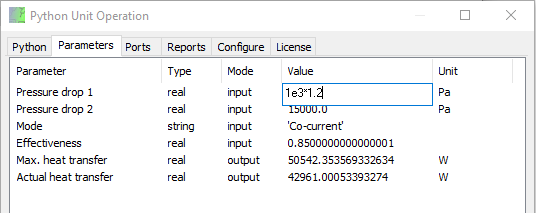In addition to `REAL` and `STRING` parameters, the Python Unit Operation supports `INTEGER`, `BOOLEAN` and `REAL_ARRAY` parameters.

### Information streams

As an alternative to parameters, one can use information streams. Most PMEs will allow Unit Operation parameters to be used in a computational manner in any case, but sometimes it makes sense to explicitly state that a specific piece of information must flow from one Unit Operation to another, and should therefore appear on an information stream. An information stream typically contains a single scalar real parameter, and that is exactly what is supported by the Python Unit Operation.

This example demonstrates a Unit Operation that measures the temperature difference between the actual stream temperature and the dew point temperature, for the purpose of exposing this information via an information stream to a controller.

#Expose temperature deviation from dew point temperature def Configure(unit): unit.add_port("Feed",unit.PortType.MATERIAL,unit.PortDirection.FEED) unit.add_port("Product",unit.PortType.MATERIAL,unit.PortDirection.PRODUCT,optional=True) unit.add_port("DewPointTemperatureDeviation",unit.PortType.INFORMATION,unit.PortDirection.PRODUCT,dimension=unit.Dimension.KELVIN,difference=True) def Calculate(unit): #copy feed to product fd=unit.ports["Feed"] if "Product" in unit.ports: #else not connected, it is optional unit.ports["Product"].copy_from(fd) #dew point dew_point_t=fd.create_phase_equilibrium(x=fd.x,p=fd.p,vaporFraction=1).t unit.ports["DewPointTemperatureDeviation"].value=fd.t-dew_point_t

Keep in mind that not all PMEs support information streams.

### Energy streams

Our simple heater in Example 1 used an `INPUT` parameter to specify the heat duty. Alternatively, an `ENERGY` port can be used.

Generic `ENERGY` energy streams transfer `work`, J/s.

An `ENERGY` `FEED` stream is an input specification. This does not mean that energy must be consumed; `work` with a negative sign means the input specification is on how much energy is produced. For a `ENERGY` `PRODUCT` stream, a positive sign means energy produced, a negative sign means energy consumed.

A `thermal=True` `ENERGY` port transfers heat. It does so within `temperature_range` which is available from a `FEED` and must be specified for a `PRODUCT`.

An `axle=True` `ENERGY` port transfers work via a rotating axle. It does so with `axis_frequency` which is available from a `FEED` and must be specified for a `PRODUCT`.

Here is a revision of Example 1, using an `ENERGY` port:

import warnings import math def Configure(unit): #add a feed and product unit.add_port("Feed",unit.PortType.MATERIAL,unit.PortDirection.FEED) unit.add_port("Product",unit.PortType.MATERIAL,unit.PortDirection.PRODUCT) #add a thermal energy inlet port WATT=unit.Dimension.KILOGRAM*unit.Dimension.METER**2/unit.Dimension.SECOND**3 unit.add_port("Heat Duty",unit.PortType.ENERGY,unit.PortDirection.FEED,thermal=True) def Calculate(unit): #copy Feed to Product, apply heat duty fd=unit.ports["Feed"] e=unit.ports["Heat Duty"] duty=e.work if fd.flow_rate==0 and duty!=0: raise RuntimeError('Zero feed flow rate; cannot apply duty') h=fd.get_property('enthalpy') #molar enthalpy if (duty!=0): h+=duty/fd.flow_rate pr=unit.ports["Product"] pr.set(x=fd.x,p=fd.p,flow_rate=fd.flow_rate,enthalpy=h) #check temperature range (t_min_e,t_max_e)=e.temperature_range if not math.isnan(t_min_e): #check against operating range t_out=pr.create_phase_equilibrium(x=fd.x,p=fd.p,enthalpy=h).t if duty>0: #heating if t_out>t_max_e: warnings.warn('heating up product stream to '+str(t_out)+' K is not feasible with heat stream with max. temperature of '+str(t_max_e)+' K') else: #cooling if t_out<t_min_e: warnings.warn('cooling down product stream to '+str(t_out)+' K is not feasible with heat stream with min. temperature of '+str(t_min_e)+' K')

Note that in addition to the heating or cooling of the product stream, the unit checks whether the temperature range on the `ENERGY` `FEED` stream is suitable for performing the heating or cooling. If the temperature range is not feasible, the unit produces a warning. Note that warnings issues via warnings.warn are automatically forwarded to the simulation environment: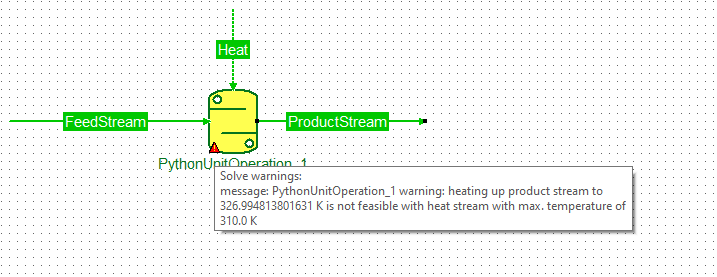An alternative arrangement is that the Product stream is heated to a specified temperature, and the required heat is reported as `ENERGY` `PRODUCT` stream.

def Configure(unit): #add a feed and product unit.add_port("Feed",unit.PortType.MATERIAL,unit.PortDirection.FEED) unit.add_port("Product",unit.PortType.MATERIAL,unit.PortDirection.PRODUCT) #outlet temperature parameter unit.add_parameter("Temperature",unit.ParameterType.REAL,unit.ParameterMode.INPUT,dimension=unit.Dimension.KELVIN) #add a thermal energy outlet port WATT=unit.Dimension.KILOGRAM*unit.Dimension.METER**2/unit.Dimension.SECOND**3 unit.add_port("Heat Duty",unit.PortType.ENERGY,unit.PortDirection.PRODUCT,thermal=True,optional=True) def Calculate(unit): #copy Feed to Product, apply temperature fd=unit.ports["Feed"] pr=unit.ports["Product"] pr.set(x=fd.x,flow_rate=fd.flow_rate,p=fd.p,t=unit.parameters['Temperature'].value) #check optional heat stream if "Heat Duty" in unit.ports: e=unit.ports["Heat Duty"] e.work=-(pr.create_phase_equilibrium(x=fd.x,p=fd.p,t=unit.parameters['Temperature'].value).get_property('enthalpy')-fd.get_property('enthalpy'))*fd.flow_rate if e.work<0: #note sign on product work #heating e.temperature_range=(fd.t,unit.parameters['Temperature'].value) else: #cooling e.temperature_range=(unit.parameters['Temperature'].value,fd.t)

### Text reports

Reports are added using `add_report` during `Configure`.

The most convenient way to write to a report is to use built-in `print` function, specifying the report in the `file` argument:

def Configure(unit): #reports unit.add_report("Test Report") def Calculate(unit): #write to report rep=unit.reports['Test Report'] print("""Lorem ipsum dolor sit amet, consectetur adipiscing elit, sed do eiusmod tempor incididunt ut labore et dolore magna aliqua. Ut enim ad minim veniam, quis nostrud exercitation ullamco laboris nisi ut aliquip ex ea commodo consequat. Duis aute irure dolor in reprehenderit in voluptate velit esse cillum dolore eu fugiat nulla pariatur. Excepteur sint occaecat cupidatat non proident, sunt in culpa qui officia deserunt mollit anim id est laborum.""",file=rep)

### Putting it all together

The last example is a bit more complicated, and uses material streams, parameters, private storage, custom validation, reporting and the abilty of displaying graphics. Here is the full listing of a multi-stream heat exchanger.

The example depends on `numpy` and `scipy` for the numerics.

In the `Configure` function, a configuration parameter is defined: `number_of_tubes`. Such a parameter cannot be exposed as input parameter, as this changes the collection of ports and parameters; CAPE-OPEN allows such collections to change only while editing the unit operation.

Subsequently, this value is stored in `private_date` so that it is available during `Validate` and `Calculate`. The value is also used during configuration; two ports, feed and product, and two parameters, UA and flow direction, are added for each tube.

The unit is allowed to connect to streams with inconsistent compound slates, `allow_inconsistent_compounds=True`, and the validation of compounds on the connected streams is analogous to Example 5 and Example 6.

To speed up the calculations, enthalpy interpolation tables are created. For the simplicity of the example, zero pressure drop is assumed, so that enthalpy is a function only of temperature, at constant composition and pressure. Enthalpy is interpolated using spline interpolation from `scipy.interpolate` for enthalpy calculated at phase equilibrium, at user specified maximum temperature intervals of `Interpolation temperature interval`. Enthalpy at phase equilibrium vs. temperature is only 0th order continuous at phase boundaries, so as to prevent improper interpolation over the phase boundaries, the dew and bubble points are found using a pressure-vapor fraction flash. If within the specified temperature region, separate interpolation tables are produced on either side of the phase boundary.

Enthalpy tables can be re-used between successive calculations, provided the overall composition did not change, the pressure did not change, and the new temperange range is within bounds of the current table. The `needs_update` method checks this. In addition, the tables should not be used if the thermodynamic configuration of any of the materials has changed. If this happens, the PME must call `Validate`.

The enthalpy tables are stored in `volatile_data`. As opposed to `private_data`, `volatile_data` is not persisted between sessions. There would be no point to save the data in persistent storage, as after restoring, `Validate` will be called as thermdynamic configuration may have changed. At `Validate`, the tables are removed from `volatile_data` using `del`.

The calculation simulates heat transer between the shell and each of the tubes, under the assumption that the heat transfer coefficients are constant. The total U*A for each tube is specified as input parameter. In addition, each tube can be co- or counter-current to the shell, which is also specified as input parameter. Discretizing the heat balances over dimensionless length leads to the following equations for cell j: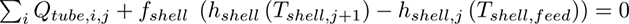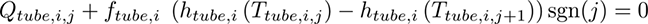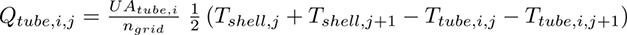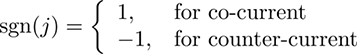Here, Q and each equation applies to a grid cell, where T applies to each grid node; the number of nodes is the number of cells plus 1. However feed temperatures are not calculated, as they are constant, and are excluded from the variables, so that the number of variables and equations match. All temperatures are organized in the direction of shell feed to shell product, so shell and co-current tubes have forward indexing with the feed temperature at the start, and counter-current tubes have reverse indexing, with feed temperature at the end. Temperature indexing is pre-calculated and stored as `t_indices` in temporary storage `stream_info`. The temperatures can then quickly be extracted in the order required for the equations, using `t_indices` on an array of `[x]+[t_feed]` where `x` is all temperatures solved for, and `t_feed` contains the constant feed temperatures.

The functions are implemented in `fun`; the Jacobian is rather sparse, but to not make the example overly complex, a dense Jacobian is implemented in `jac`. The equations are solved using `scipy.optimize.root` with a tolerance that the user provides as input parameter. Note that this tolerance, applies only to solving the equations. Some additional systemic error rises from the use of interpolation tables. This error can be reduced by choosing a smaller value for `Interpolation temperature interval`.

Any profiles stored from an initial guess can of course be used as an initial guess. The solution therefore proceeds in two attempts, due to the for loop over `use_initial_guess`. In the first attempt, if profiles are available, and if the shape of the profiles (number of tubes, number of grid cells) match the current problem, the initial guess is backed out from the stored profiles.

If this fails, or if appropriate profiles are not available, an initial guess is calculated. In the special case that all tubes are co-current with the shell, the initial guess exit temperatures are solved as one common temperature for which the heat balance closes. This temperature is bracketed between the min. and max. value of all inlet temperatures; these equations are implemented in `f_heat_balance` and solved using `scipy.optimize.toms748`.

If at least one tube if counter-current, a rough initial guess is calculated by solving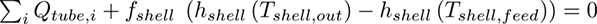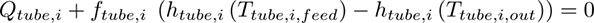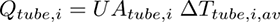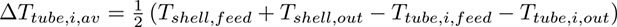The equations are implemented in `f_init` along with the full Jacobian, and solved using `scipy.optimize.root`.

After estimating the outlet temperatures, the initial guess is constructed from linear interpolation between inlet and outlet temperatures.

The `WriteProfileReport` demonstrates how to use the `print` statement with the `file` argument set to a report; `WriteProfileReport` is called with `unit.reports['Temperature profiles']` as argument. The reports are not only exposed to the PME, but also shown in the Reports tab: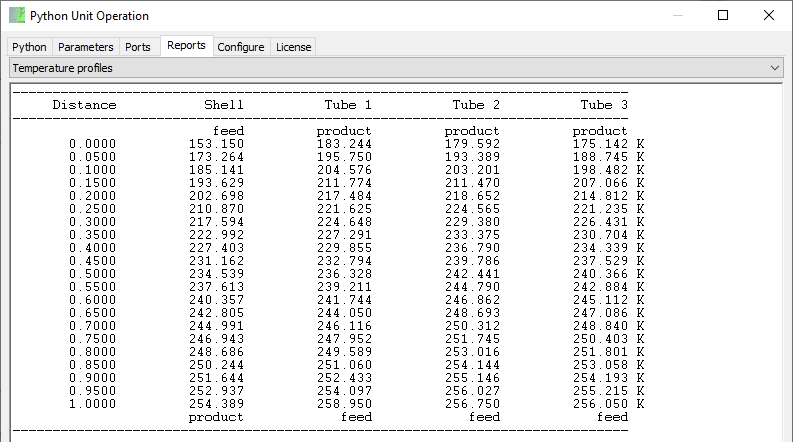Finally, `matplotlib` is used to create a graph showing the temperature profiles as a function of dimensionless distance. Images can be added just like reports, using `add_image` in `Configure`. The script must supply a function that creates a PNG file for the image at requested width and height, see `CreateTemperatureGraph`. Note that, in contrast to creation of reports, images are not created as part of `Calculate`, but on request, with specified size. The unit should save relevant data to create the image. The image is displayed on the Report tab of the edit window: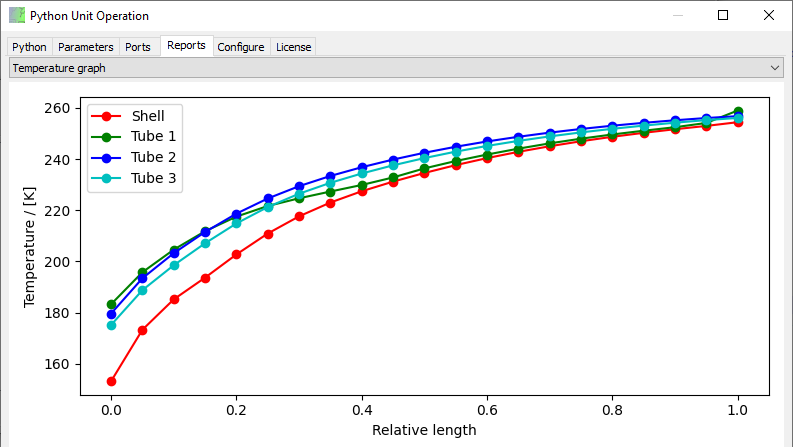### Building a library

So far, the module that defines the Unit Operation, its configuration and its calculation, has been typed directly in the editor. This script will be saved as part of the Unit Operation.

Sometimes you want the same Unit Operation definition to be used in multiple places. One way to do this is to copy and paste the script. Which works fine. But it is not very maintenance friendly. If you discover a bug in the script, or want to modify the calculation, all Unit Operations that are defined by the same script must be edited separately to correct for the same issues.

As an alternative, the Unit Operation can be defined from an external module. To demonstrate how this works, the following steps are followed.

• This example assumes that the library of Unit Operation definitions exists in a folder `Python Unit Operation Models`, and that this folder is a sub-folder of the `My Documents` folder. Create this folder,
• Copy the Multi-stream shell and tube heat exchanger code from Example 12, and place it in a file `multistreamhtx.py`, in the `Python Unit Operation Models` folder,
• Define the Unit Operation from Example 13.
import os import sys def GetDocumentFolder(): #returns the My Documents folder for the current user import ctypes.wintypes pathbuffer=ctypes.create_unicode_buffer(ctypes.wintypes.MAX_PATH) ctypes.windll.shell32.SHGetFolderPathW(None,5,None,0,pathbuffer) return pathbuffer.value def GetUnitOperationModelFolder(): #assumes the My Documents folder has a sub-folder Python Unit Operation Models return os.path.join(GetDocumentFolder(),"Python Unit Operation Models") def Configure(unit): sys.path.append(GetUnitOperationModelFolder()) #add Python Unit Operation Models to the path import multistreamhtx #load the module of interest unit.define_from(multistreamhtx) #define the unit operation from this module

Of course if the folder that contains the Unit Operation models is already in the Python path, the script becomes a lot simpler:

import multistreamhtx def Configure(unit): unit.define_from(multistreamhtx)